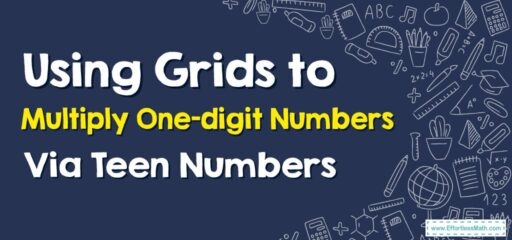# How to Use Grids to Multiply One-digit Numbers By Teen Numbers

Using a grid to multiply one-digit numbers by teen numbers can be a useful way to visualize the problem and make it easier to solve.## A Step-by-step Guide to Using Grids to Multiply One-digit Numbers By Teen Numbers

Of course, let’s break down how we can multiply one-digit numbers by teen numbers using a grid.

Here’s an example: Let’s multiply $$4 x 13$$.

### Step 1: Break Down the Teen Number

Teen numbers are numbers from 10 to 19. They can be split into 10 and a single-digit number. In our example, 13 can be broken down into 10 and 3.

### Step 2: Draw the Grid

Draw a simple 2-row grid. The rows will represent the two parts of the teen number (10 and 3), and the single column will represent the one-digit number (4).

   | 4
-----------
10 |
-----------
3  |


### Step 3: Multiply

Now, multiply the number in each row by the number in the column. So, you’ll multiply 4 by 10 and 4 by 3.

   |  4
-----------
10 | 40
-----------
3  | 12


### Step 4: Add the Results

Now, add the results of the two multiplications together. In this case, $$40 + 12 = 52$$.

So, $$4 x 13 = 52$$.

This grid method is a helpful way to understand multiplication with teen numbers, as it breaks the process down into simpler steps. It’s particularly useful for visual learners and those who might struggle with traditional multiplication methods.

### What people say about "How to Use Grids to Multiply One-digit Numbers By Teen Numbers - Effortless Math: We Help Students Learn to LOVE Mathematics"?

No one replied yet.

X
30% OFF

Limited time only!

Save Over 30%

SAVE $5 It was$16.99 now it is \$11.99